Open In App
Related Articles
• Digital Electronics and Logic Design Tutorials

# Logic Gates – Definition, Types, Uses

A semiconductor material’s electrical conductivity is somewhere between that of a conductor, such as metallic copper, and that of an insulator, such as glass. As the temperature rises, its resistivity reduces, whereas metals have the opposite effect. The conductivity of a crystal structure can be modified in a favorable way by introducing impurities (doping) to it. When two separate doped regions in the same crystal exist, a semiconductor junction is generated. The behavior of charge carriers such as electrons, ions, and electron holes at these junctions is the foundation of diodes, transistors, and most modern electronics.

Semiconductors include silicon, germanium, gallium arsenide, and elements on the periodic table’s so-called metalloid staircase. After silicon, gallium arsenide is the second most common semiconductor, and it’s used in laser diodes, solar cells, microwave-frequency integrated circuits, and other things. Silicon is a critical component in the manufacture of nearly all electrical circuits.

## Logic Gates

A logic gate is a simple switching circuit that determines whether an input pulse can pass through to the output in digital circuits.

The building blocks of a digital circuit are logic gates, which execute numerous logical operations that are required by any digital circuit. These can take two or more inputs but only produce one output.

The mix of inputs applied across a logic gate determines its output. Logic gates use Boolean algebra to execute logical processes. Logic gates are found in nearly every digital gadget we use on a regular basis. Logic gates are used in the architecture of our telephones, laptops, tablets, and memory devices.

## Boolean Algebra

Boolean algebra is a type of logical algebra in which symbols represent logic levels.

The digits(or symbols) 1 and 0 are related to the logic levels in this algebra; in electrical circuits, logic 1 will represent a closed switch, a high voltage, or a device’s “on” state. An open switch, low voltage, or “off” state of the device will be represented by logic 0.

At any one time, a digital device will be in one of these two binary situations. A light bulb can be used to demonstrate the operation of a logic gate. When logic 0 is supplied to the switch, it is turned off, and the bulb does not light up. The switch is in an ON state when logic 1 is applied, and the bulb would light up. In integrated circuits (IC), logic gates are widely employed.

Truth Table: The outputs for all conceivable combinations of inputs that may be applied to a logic gate or circuit are listed in a truth table. When we enter values into a truth table, we usually express them as 1 or 0, with 1 denoting True logic and 0 denoting False logic.

## Types of Logic Gates

A logic gate is a digital gate that allows data to be transferred. Logic gates, use logic to determine whether or not to pass a signal. Logic gates, on the other hand, govern the flow of information based on a set of rules. The following types of logic gates are commonly used:

1. AND
2. OR
3. NOT
4. NOR
5. NAND
6. XOR
7. XNOR

## Basic Logic Gates

### AND Gate

An AND gate has a single output and two or more inputs.

1. When all of the inputs are 1, the output of this gate is 1.
2. The AND gate’s Boolean logic is Y=A.B if there are two inputs A and B.

An AND gate’s symbol and truth table are as follows: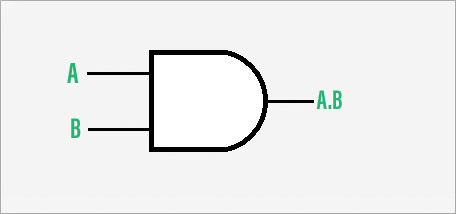Symbol of AND gate

Therefore, in And gate, the output is high when all the inputs are high.

### OR Gate

Two or more inputs and one output can be used in an OR gate.

1. The logic of this gate is that if at least one of the inputs is 1, the output will be 1.
2. The OR gate’s output will be given by the following mathematical procedure if there are two inputs A and B: Y=A+B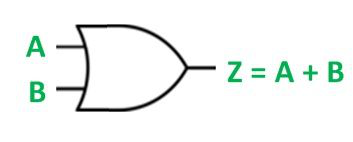Symbol of OR gate

Therefore, in the OR gate, the output is high when any of the inputs is high.

### NOT Gate

The NOT gate is a basic one-input, one-output gate.

1. When the input is 1, the output is 0, and vice versa. A NOT gate is sometimes called an inverter because of its feature.
2. If there is only one input A, the output may be calculated using the Boolean equation Y=A’.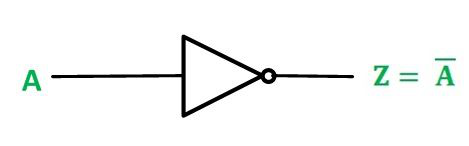Symbol of NOT gate

A NOT gate, as its truth table shows, reverses the input signal.

## Universal Logic Gates

### NOR Gate

A NOR gate, sometimes known as a “NOT-OR” gate, consists of an OR gate followed by a NOT gate.

1. This gate’s output is 1 only when all of its inputs are 0. Alternatively, when all of the inputs are low, the output is high.
2. The Boolean statement for the NOR gate is Y=(A+B)’ if there are two inputs A and B.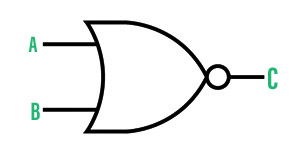Symbol of NOR gate

By comparing the truth tables, we can observe that the outputs of the NOR gate are the polar opposite of those of an OR gate. The NOR gate is sometimes known as a universal gate since it may be used to implement the OR, AND, and NOT gates.

### NAND Gate

A NAND gate, sometimes known as a ‘NOT-AND’ gate, is essentially a Not gate followed by an AND gate.

1. This gate’s output is 0 only if none of the inputs is 0. Alternatively, when all of the inputs are not high and at least one is low, the output is high.
2. If there are two inputs A and B, the Boolean expression for the NAND gate is Y=(A.B)’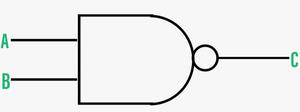Symbol of NAND gate

By comparing their truth tables, we can observe that their outputs are the polar opposite of an AND gate. The NAND gate is known as a universal gate because it may be used to implement the AND, OR, and NOT gates.

## Other Logic Gates

### XOR Gate

The Exclusive-OR or ‘Ex-OR’ gate is a digital logic gate that accepts more than two inputs but only outputs one value.

1. If any of the inputs is ‘High,’ the output of the XOR Gate is ‘High.’ If both inputs are ‘High,’ the output is ‘Low.’ If both inputs are ‘Low,’ the output is ‘Low.’
2. The Boolean equation for the XOR gate is Y=A’.B+A.B’ if there are two inputs A and B.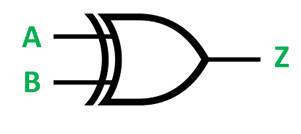Symbol of XOR gate

Its outputs are based on OR gate logic, as we can see from the truth table.

### XNOR Gate

The Exclusive-NOR or ‘EX-NOR’ gate is a digital logic gate that accepts more than two inputs but only outputs one.

1. If both inputs are ‘High,’ the output of the XNOR Gate is ‘High.’ If both inputs are ‘Low,’ the output is ‘High.’ If one of the inputs is ‘Low,’ the output is ‘Low.’
2. If there are two inputs A and B, then the XNOR gate’s Boolean equation is: Y=A.B+A’B’.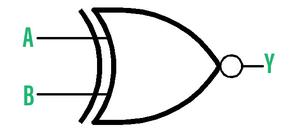Symbol of XNOR gate

The truth table shows that its outputs are based on NOR gate logic.

## Uses of Logic Gates

1. Logic gates are utilized in a variety of technologies. These are components of chips (ICs), which are components of computers, phones, laptops, and other electronic devices.
2. Logic gates may be combined in a variety of ways, and a million of these combinations are necessary to make the newest gadgets, satellites, and even robots.
3. Simple logic gate combinations can also be found in burglar alarms, buzzers, switches, and street lights. Because these gates can make a choice to start or stop based on logic, they are often used in a variety of sectors.
4. Logic gates are also important in data transport, calculation, and data processing. Even transistor-transistor logic and CMOS circuitry make extensive use of logic gates.

## Solved Examples on Logic Gates – Definition, Types, Uses

Question 1: What are Logic gates?

Logic gates are digital circuits that conduct logical operations on the input provided to them and produce appropriate output.

Question 2: What are Universal gates?

To accomplish a specific logical process, universal gates are created by merging two or more fundamental gates. Universal gates are NAND and NOR gates.

Question 3: What is the output of a NOT gate when input 0 is applied?

Because NOT gate is an inverter. As a result, if 0 is used as an input, the output will be 1.

Question 4:  Which logic gate is known as the “invertor”?

An invertor is also known as a NOT gate. The obtained output is the inverse of the input.

Question 5:  What is the Boolean expression for OR gate?